• ## 匹配滤波

千次阅读 2020-09-23 20:45:27
匹配滤波（matched filtering）是最佳滤波的一种。当输入信号具有某一特殊波形时，其输出达到最大。在形式上，一个匹配滤波器由以按时间反序排列的输入信号构成。且滤波器的振幅特性与信号的振幅谱一致。因此，对...
    匹配滤波（matched filtering）是最佳滤波的一种。当输入信号具有某一特殊波形时，其输出达到最大。在形式上，一个匹配滤波器由以按时间反序排列的输入信号构成。且滤波器的振幅特性与信号的振幅谱一致。因此，对信号的匹配滤波相当于对信号进行自相关运算。配滤波器是一种非常重要的滤波器，广泛应用与通信、雷达等系统中。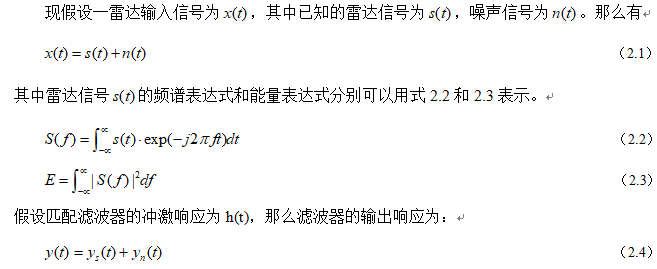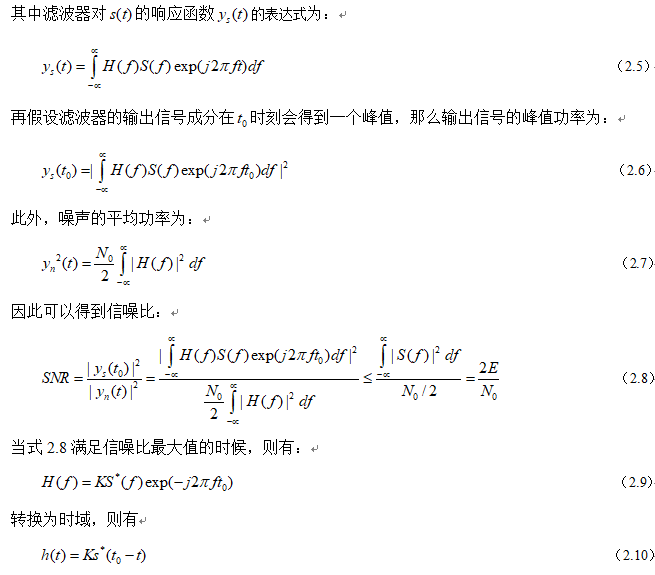从上面的理论推导可以看到，当输出信噪比为最大值的时候，滤波器的传递函数与输入信号的频谱函数满足特定的关系，式2.10就反映了这个关系。满足这种关系的线性滤波器，称之为匹配滤波器。

匹配滤波器的在数学上的运算作用就是对输入信号s(t)做相关运算。在时刻，信号各频率分量同相叠加，得到最大输出值，其输出值的大小只与信号能量有关。

通过上面的分析可知，所谓的最优滤波器，实际上都是在某个准则下的最优。匹配滤波器对应的最优的准则是输出信噪比(SNR)最大。而且还有一个前提条件是在白噪声背景下。 匹配滤波器在很多场合有应用，本课题的雷达信号脉冲压缩技术中匹配滤波器是一个核心功能模块，在MATLAB中仿真，可以得到匹配滤波前后的仿真效果图如图2.1所示。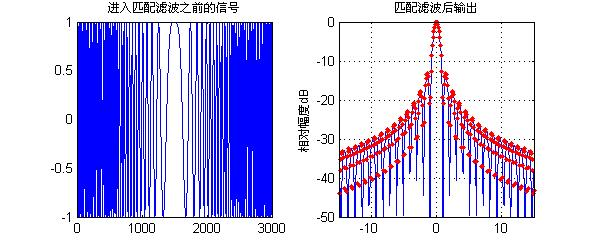图2.1 雷达信号进入匹配滤波器前后对比仿真图

从上面的仿真可以看到，当信号通过匹配滤波器之后，信号的在某一时刻会产生一个最大值，这个最大值就是滤波器的输出值，其胖瓣会产生明显的衰减现象。通过匹配滤波之后的雷达信号，由于对其进行目标的跟踪与搜索。

通过上面的理论分析和仿真可以知道，匹配滤波器具备如下的特征：

一方面，从幅频特性来看，匹配滤波器和输入信号的幅频特性完全一样。这也就是说，在信号越强的频率点，滤波器的放大倍数也越大；在信号越弱的频率点，滤波器的放大倍数也越小。也就是说，匹配滤波器是让信号尽可能通过，而不管噪声的特性。

另外一方面，从相频特性上看，匹配滤波器的相频特性和输入信号正好完全相反。这样，通过匹配滤波器后，信号的相位为0，正好能实现信号时域上的相干叠加。而噪声的相位是随机的，只能实现非相干叠加。这样在时域上保证了输出信噪比的最大。

实际上，在信号与系统的幅频特性与相频特性中，幅频特性更多地表征了频率特性，而相频特性更多地表征了时间特性。匹配滤波器无论是从时域还是从频域，都充分保证了信号尽可能大地通过，噪声尽可能小地通过，因此能获得最大信噪比的输出。

展开全文• 运用matlab实现匹配滤波，文件中有匹配滤波的文档说明matlab
• 包含多种匹配滤波方法的设计，以及QPSK、MFSK、QAM的应用工程。
• 线性调频波LFM是雷达成像的发射信号，本程序含有LFM的回波生成以及LFM的匹配滤波，最后给出图像
• 成型滤波与匹配滤波总是成对出现。常见的组合有 升余弦滤波（发射端）+ 单位冲击响应（接收端） 平方根升余弦滤波（发射端）+ 平方根升余弦滤波（接收端） 成型滤波的波形不只升余弦的方式，还有其他波形。后面...
成型滤波与匹配滤波总是成对出现。常见的组合有

升余弦滤波（发射端）+ 单位冲击响应（接收端）
平方根升余弦滤波（发射端）+ 平方根升余弦滤波（接收端）

成型滤波的波形不只升余弦的方式，还有其他波形，可以通过眼图可以查看区别。后面补充成型滤波设计原理和作用原理。
MATLAB设计
b = rcosdesign(beta,span,sps,shape);

beta——滚降系数
span——截断符号数
sps——过采样倍数
shape——‘sqrt’表平方根升余弦滤波器，‘normal’为升余弦滤波器。


展开全文• 本程序主要是对雷达信号进行匹配滤波，提高信噪比，还有对LFM信号设计。确保运行。
• 本文分析了纯相位匹配滤波实现特征识别的物理原因。据此我们修改了待识别物体的傅里叶变换的振幅,以减小光强度的动态范围。同时,保持其傅里叶变换的相位不变。用重铬酸盐明胶记录反射式全息匹配滤波器,从而获得高...
•研究论文
• 以远程脉冲激光外差探测系统为模型, 推导了系统的信号与噪声的表达式, 给出了脉冲激光外差探测系统匹配滤波算法流程, 对匹配滤波技术在中程导弹与国际空间站目标探测中的详细应用过程进行了仿真, 分析了目标距离、...
• 设计了一种多芯光纤Bragg光栅曲率传感器, 并采用匹配滤波技术实现曲率解调。多芯光纤Bragg光栅曲率传感器是通过在多芯光纤的两个中心对称纤芯中写入Bragg光栅实现的。两个光纤Bragg光栅具有相似的反射谱和中心波长, ...
• 本文研究了振幅补偿匹配滤波的旋转效应和尺度变化效应.计算机模拟的结果表明,这种滤波法对输入信号的旋转变化和尺度缩放变化,相对于传统的匹配滤波法及仅相位滤波法要敏感得多.文中还给出了这三和滤波下的相关峰值与...
• 概述性描述匹配滤波在气象雷达中应用，介绍了匹配滤波原理算法软硬件实现
•研究论文
• 匹配滤波源码，详情见http://blog.csdn.net/u013288466/article/details/66473284
• 一，匹配滤波原因 匹配滤波又叫去斜处理，去斜处理“有源相关”，通常用来处理极大带宽的LFM波形 （如果直接采样的话因为频带很宽所以在高频的时候需要的采样率就很大，采样点数就很多，所以要经过去斜处理）。 ...
一，匹配滤波原因

匹配滤波又叫去斜处理，去斜处理“有源相关”，通常用来处理极大带宽的LFM波形

（如果直接采样的话因为频带很宽所以在高频的时候需要的采样率就很大，采样点数就很多，所以要经过去斜处理）。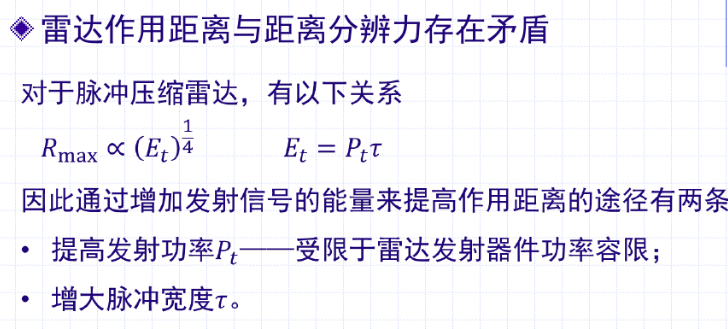为了解决传统单频脉冲面临的作用距离和空间分辨率之间的矛盾，脉冲压缩技术一般采用：

发射宽度相对较宽而峰值功率低的脉冲，使信号有足够的能量以保证作用距离；

接收时做匹配滤波，将底峰值的宽脉冲压缩成高峰值的窄脉冲，避免脉冲重叠现象，从而提高空间分辨率。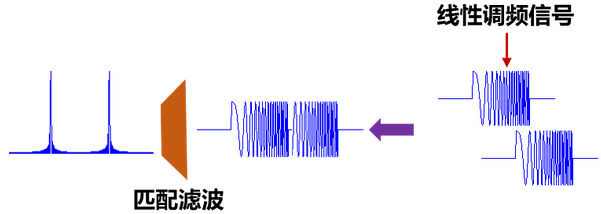二，匹配滤波

匹配滤波原理：匹配滤波器（match filter）是最佳线性滤波器的一种，该滤波器的准则是输出信噪比最大，常用于通信、雷达等系统的接收机中，下面对其冲激响应/系统函数进行推导。
设该滤波器传递函数为H(f)H(f)，冲激响应为h(t)h(t)，输入信号为r(t)=s(t)+n(t)，其中s(t)为输入信号，n(t)为高斯白噪声。设输入信号的频谱密度函数为S(f)，而高斯白噪声的单边功率谱为n0/2，其中n0为高斯白噪声单边功率谱密度。

其中：S(t)=Acos(2πf_0 t+πμt^2) (0≤t≤τ)
h(t)=ks_t^ (t_0-t)
H(f)=kS_t^ (f)e^(-j2πft_0 )
τ^’=1/B=1/μτ**在通信系统中，滤波器是其中重要部件之一，滤波器特性选择直接影响数字信号的恢复。在数字信号接收中，滤波器的作用有两个方面，使滤波器输出有用信号成分尽可能强；抑制信号外带噪声，使滤波器输出噪声成分尽可能小，减少噪声对信号判决的影响。对最佳线形滤波器的设计有两种准则其中一种是是滤波器输出信噪比在某一特定时刻达到最大，由此而导出的最佳线性滤波器成为匹配滤波器。在数字通信中，匹配滤波器具有广泛的应用。因此匹配滤波器是指滤波器的性能与信号的特性取得某种一致，使滤波器输出端的信号瞬时功率与噪声平均功率的比值最大。在信号处理中，匹配滤波器可以用来解调基频带脉冲信号，基频带脉冲信号意指信号内容为同一波形信号乘上一个常数，在每个周期出现，每个周期中代表着或多或少的信息量。匹配滤波器解调出来的结果其SNR(Signal Noise Ratio)为最大的，匹配滤波器需要事先知道：1.传送的信号；2.信号的同步。这样才能解调出传送的信号。

三，去斜处理
Stretch方法是针对线性调频信号而提出的，其方法是将输入信号与参考信号（经适当延迟的本振信号，延迟量通常由窄带信号测距结果估计出）混频，则每一个散射点就对应一个混频后的单频分量，对混频输出的信号进行DFT处理，即可获得目标的距离像，对参考信号的要求是应具有与输入信号相同的调频斜率。混频过程为回波信号在时域与参考信号的共轭相乘

混频后得到一个瞬时频率和目标距离成正比的单频信号，对其进行频谱分析即可得到目标的距离像；去斜处理一般情况下可降低信号带宽；

1，生成输入信号和参考信号

%%%%%%%%%%%%%%%%%%%%%%%%  去斜处理仿真程序  %%%%%%%%%%%%%%%%%%%%%%%%%
clc;clear all;close all;
B=10e6;%带宽10MHz
tp=10e-6;%脉宽10us
k=B/tp;%LFM系数
fs=50e6;
R0=3e3;R1=2000;R2=3500;R=5000;
c=3e8;
f0=60e6;

N=round(2*R/c*fs);
fft_N=2^nextpow2(N);
t=linspace(0,2*R/c,N);

%%%%%%%%%%%%%%%%%%%%%%%%%%   参考信号   %%%%%%%%%%%%%%%%%%%%%%%%%%%%%%
Sref=exp(2i*pi*f0*t).*exp(1i*pi*k*t.^2);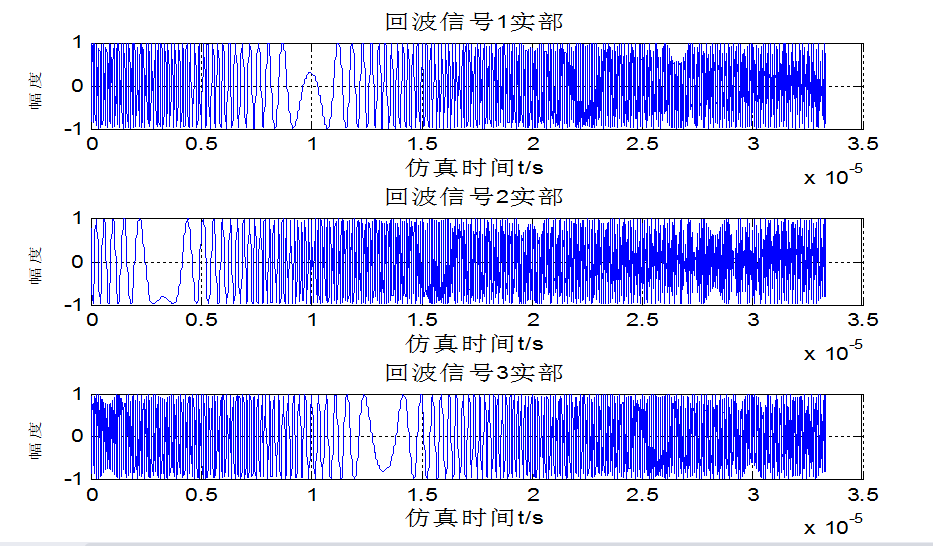2，生成回波信号

%%%%%%%%%%%%%%%%%%%%%%%%%%%   回波信号   %%%%%%%%%%%%%%%%%%%%%%%%%%%%
Sb0=exp(1j*pi*k*(t-2*R0/c).^2).*exp(2j*pi*f0*(t-2*R0/c));
Sb1=exp(1j*pi*k*(t-2*R1/c).^2).*exp(2j*pi*f0*(t-2*R1/c));
Sb2=exp(1j*pi*k*(t-2*R2/c).^2).*exp(2j*pi*f0*(t-2*R2/c));
Sb=Sb0+Sb1+Sb2;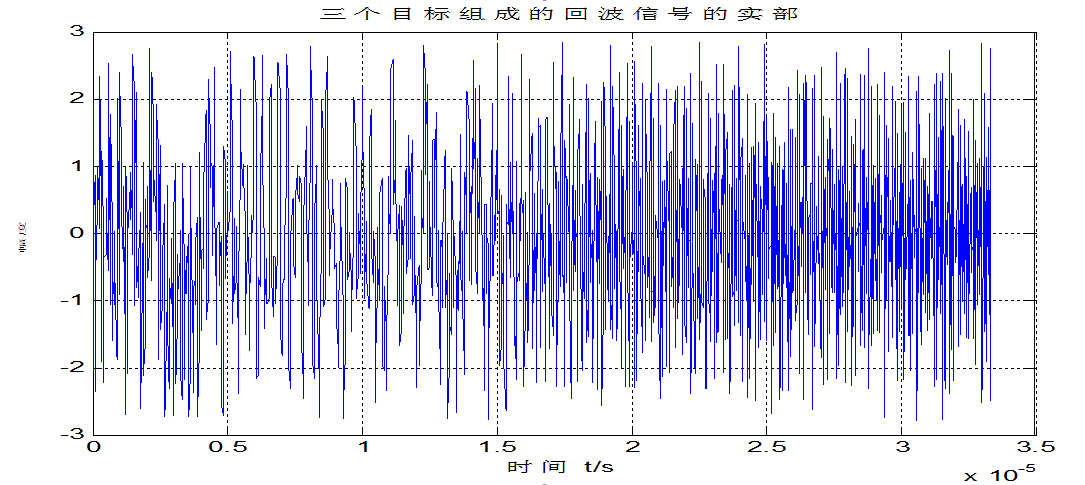3，混频信号

%%%%%%%%%%%%%%%%%%%%%%%%%%%   混频信号   %%%%%%%%%%%%%%%%%%%%%%%%%%%%
SSb=Sref.*conj(Sb);%去斜后时域信号
spectrum=fft(SSb,fft_N);%去斜后频域信号
f=fs*(0:fft_N-1)/fft_N-fs/2;%从-fs/2到fs/2
f=f*c*tp/2/B;%瞬时频率对应的距离
sf=exp(-j*pi/k*f.^2);%滤波器传输函数
SSb=spectrum.*sf;%从频域去距离扭曲，实现了压缩和去RVP
figure;
SSb=fftshift(SSb);
SSb1=ifft(SSb);%消除了距离扭曲和RVP的时域信号
subplot(211);
plot(f,db(abs(SSb)/max(SSb)))
xlabel('距离/m');
grid on
subplot(212);
plot(f,abs(SSb))
xlabel('距离/m');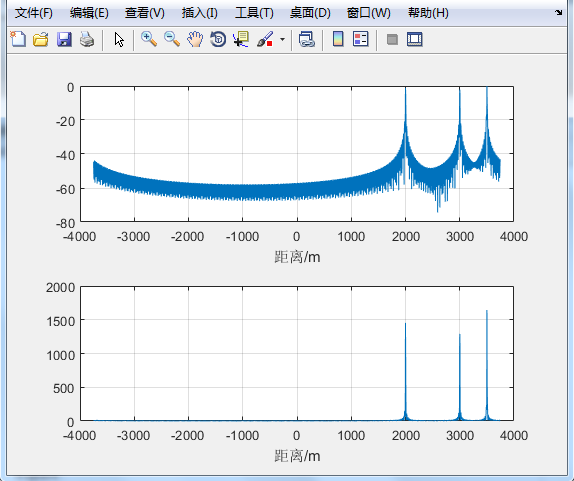展开全文• 提出了用逆滤波器来进行旋转不变匹配滤波相关识别方法;采用级联变形滤波器可以实现透射匹配相关峰强度对透射输入能量的优化,大大改善相关峰的锐度,计算机模拟结果显示,对旋转图像,在平面内按0°～32°旋转,每4°取一...
• 提出了一种合成孔径激光成像雷达（SAIL）的二维匹配滤波成像算法，对利用单频本振激光与线性调频信号光外差接收得到的SAIL目标回波信号同时在距离向、方位向进行相位二次项匹配滤波以实现目标成像。给出了单频本振...
• 1 基本概念 互相关 对于两个连续信号f和g，以g为参考，互相关的定义公式为： 卷积 ...即以信号g为参考，f与g的互相关，其频率域结果是信号g的频谱的共轭乘以f的频谱。...脉冲压缩：匹配滤波+窗函数设计抑制旁瓣 ...
1 基本概念

互相关

对于两个连续信号f和g，以g为参考，互相关的定义公式为：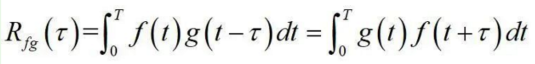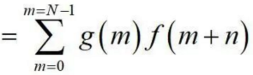相关的性质

共轭对称性；
自相关函数在原点的值等于信号能量；
相关函数的面积等于信号面积模的平方；
复信号s(t)自相关函数的傅里叶变换是正实函数；如果二个信号在频域上具有相同的能谱，在时域上具有不同的波形，但是这二个信号的相关函数却相同。

相关系数：考察两个事物（在数据里我们称之为变量）之间的相关程度。

如果有两个变量：X、Y，最终计算出的相关系数的含义可以有如下理解：

(1)、当相关系数为0时，X和Y两变量无关系。

(2)、当X的值增大（减小），Y值增大（减小），两个变量为正相关，相关系数在0.00与1.00之间。

(3)、当X的值增大（减小），Y值减小（增大），两个变量为负相关，相关系数在-1.00与0.00之间。

相关系数的绝对值越大，相关性越强，相关系数越接近于1或-1，相关度越强，相关系数越接近于0，相关度越弱。

通常情况下通过以下取值范围判断变量的相关强度：

相关系数     0.8-1.0     极强相关                 0.6-0.8     强相关                 0.4-0.6     中等程度相关                 0.2-0.4     弱相关                 0.0-0.2     极弱相关或无相关

卷积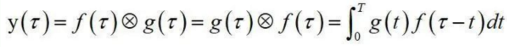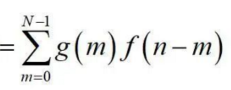相关和卷积的关系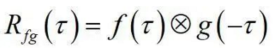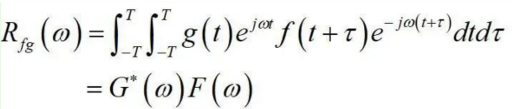即以信号g为参考，f与g的互相关，其频率域结果是信号g的频谱的共轭乘以f的频谱。

相关与卷积的区别

相关运算中被积函数没有时间反褶的过程，而卷积运算中有。
相关函数不满足交换，而卷积可以。
相关公式和卷积公式很像，相关能利用卷积表示，所以有人就觉得两个概念有关系，其实二者从概念上没有联系。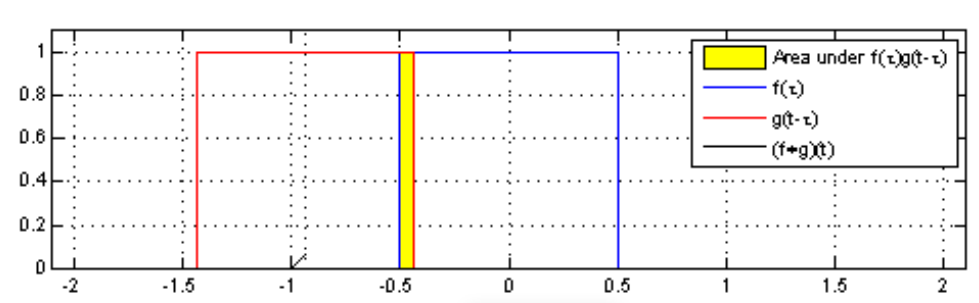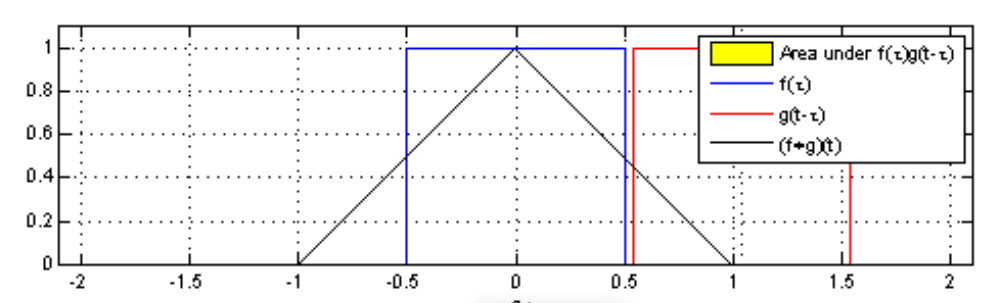匹配滤波

以发射信号g为参考，回波f和发射信号g做相关。

对于线性时不变系统，时域上，输出为输入与系统函数的卷积y(t)=g(t)*h(t)；频域上，输出为输入与传递函数的乘积Y(ω)=G(ω)×H(ω)。

如果接收机与接收到的信号匹配，那么接收机的传递函数将是输入端接收信号的复共轭    H(ω)=G* (ω)    则 Y(ω)=F(ω)×G* (ω)频域中函数的复共轭等于时域中对应信号的时间反转h(t)=G* (-t)。因此有

所以匹配滤波器是最大输出信噪声比意义下的最佳线性滤波器。 用匹配滤波器构成的接收机是满足最大输出信噪比准则的最佳接收机， 也称为匹配滤波器接收机。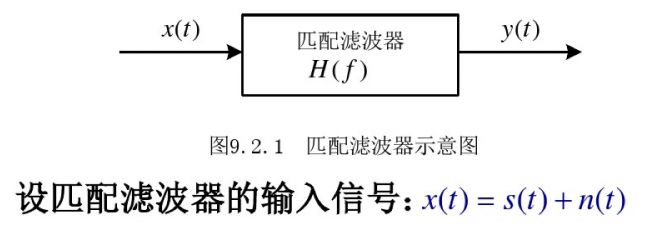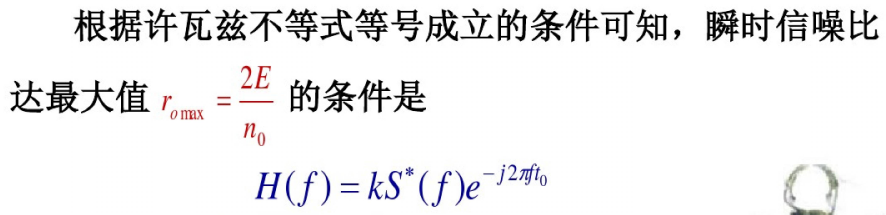上式为自相关函数的等价形式，因此，匹配滤波器冲激响应与输入信号的卷积等于输入信号的自相关函数。

脉冲压缩：匹配滤波+窗函数设计抑制旁瓣

数字脉冲压缩系统首先要将回波信号经 A/D 采样变成数字信号，再进行脉冲压缩。
数字时域脉冲压缩实际上是将回波数据与匹配滤波器进行卷积运算。 而数字频域脉冲压缩则是通过对回波数据进行 FFT 处理后， 在频域与匹配滤波器的系数进行
复数乘法运算，然后再经过 IFFT 得到压缩脉冲的结果， 尤其在采集点数也即一次运算点数较多的情况下， 其减少了运算量。

coeff = conj(fliplr(sendsignal)); %翻转共轭 send_signal_tre
fft_n = length(wave_01);     %coeff  length(echo_signal_cut); 2^(floor(log2(length(echo_signal_cut))) + 1);
output = ifft(fft(wave_01,fft_n).*fft(coeff,fft_n)); % 未截取不完全滤波点
output1 = conv(wave_01,coeff);

close all;
clear all;
% ========== 线性调频信号数字化正交解调 ========================
% ========= 产生线性调频信号 ===================
t=10e-6;                       % 信号的时间长度， 单位s
fs=30e6;                        % 采样频率，单位Hz
ts=1/fs;
fc=5e6;                         % 线性调频信号的起始频率
f0=7.5e6;                        % 本振信号的中心频率
B=5e6;                          % 调频带宽
ft=0:1/fs:t-1/fs;               % 时间序列步长
N=length(ft);
k=B/fs*2*pi/max(ft);          % modulate 函数产生
y=modulate(ft,fc,fs,'fm',k);

%k=B/t;                         % 直接公式产生
%y=cos(2*pi*(f0*ft+k*ft.^2/2));
y_fft_result=fft(y);
figure,subplot(2,1,1),plot(ft,y),xlabel('t(单位：秒)'),ylabel('y(单位：伏)'),title('线性调频信号y(t)');
subplot(2,1,2),plot((0:fs/N:fs/2-fs/N),10*log(abs(y_fft_result(1:N/2)))),xlabel('频率f(单位：Hz)'),title('线性调频信号y(t)的频谱');

% ========= 正交解调 ============================
n=0:N-1;
local_oscillator_i=cos(n*f0/fs*2*pi);       % i 路本振
local_oscillator_q=sin(n*f0/fs*2*pi);       % q 路本振
fbb_i=local_oscillator_i.*y;                % i 路解调
fbb_q=local_oscillator_q.*y;                % q 路解调
window=chebwin(51,40);                      % 设计50阶cheby窗的滤波器
[b,a]=fir1(50,2*B/fs,window);

fbb_i=[fbb_i,zeros(1,25)];
% fir 滤波器有25个采样周期延迟，为保证所有信息全部通过滤波器，故补充25个0
fbb_q=[fbb_q,zeros(1,25)];

fbb_i=filter(b,a,fbb_i);                    % 低通滤波
fbb_q=filter(b,a,fbb_q);

fbb_i=fbb_i(26:end);
fbb_q=fbb_q(26:end);

figure,subplot(2,1,1),plot(ft,fbb_i),xlabel('t(单位：秒)'),title('解调后的I路信号');
subplot(2,1,2),plot(ft,fbb_q),xlabel('t(单位：秒)'),title('解调后的Q路信号');

fbb=fbb_i+j*fbb_q;
fbb_fft_result=fft(fbb);
figure,plot((0:fs/N:fs/2-fs/N),10*log(abs(fbb_fft_result(1:N/2)))),xlabel('频率f(单位：Hz)'),title('解调后信号的频谱');

%%%%%%%%%%% =========== 产生理想线性调频脉冲压缩系数 ==========================

M=4096;
D=B*t;
match_filter=ts*fliplr(conj(fbb))*sqrt(D)*2/t;
%加窗处理
w=hamming(300);
w=w';
w_match_filter=match_filter.*w;
w_match_filter_fft=fft(w_match_filter,M);
%未加窗处理

match_filter_fft=fft(match_filter,M);

figure,subplot(2,1,1),plot(real(match_filter_fft)),title('脉冲压缩系数（实部）');
subplot(2,1,2),plot(imag(match_filter_fft)),title('脉冲压缩系数（虚部）');

figure,subplot(2,1,1);plot(10*log(abs(match_filter_fft)));subplot(2,1,2);plot(match_filter);

% ================== 产生理想回波信号 ========================

t1=100e-6;
% signal=[zeros(1,(t1-2*t)/ts),y,zeros(1,t/ts)];      % 在13.5km处产生一个点目标
% signal=[zeros(1,(t1-4*t)/ts),y,zeros(1,t/ts),y,zeros(1,t/ts)];      % 产生2个独立点目标
% signal=[zeros(1,(t1-3*t)/ts),y,y,zeros(1,t/ts)];      % 产生2个相邻点目标

signal1=[zeros(1,(t1-2*t)/ts),y,zeros(1,t/ts)];      % 产生2个重叠点目标
signal2=[zeros(1,(t1-2.2*t)/ts),y,zeros(1,1.2*t/ts)];      % 产生2个重叠点目标
signal=signal1+signal2;
n=1:1:length(signal);%4000;
figure,subplot(2,1,1),plot(n,signal1,n,signal2);
subplot(2,1,2),plot(signal),title('发射的LFM信号');

n=0:t1/ts-1;

local_oscillator_i=cos(n*f0/fs*2*pi);       % i 路本振
local_oscillator_q=sin(n*f0/fs*2*pi);       % q 路本振
fbb_i=local_oscillator_i.*signal;                % i 路解调
fbb_q=local_oscillator_q.*signal;                % q 路解调
window=chebwin(51,40);                      % 设计50阶cheby窗的滤波器
[b,a]=fir1(50,2*B/fs,window);

fbb_i=[fbb_i,zeros(1,25)];                  % fir 滤波器有25个采样周期延迟，为保证所有信息全部通过滤波器，故补充25个0
fbb_q=[fbb_q,zeros(1,25)];

fbb_i=filter(b,a,fbb_i);                    % 低通滤波
fbb_q=filter(b,a,fbb_q);

fbb_i=fbb_i(26:end);
fbb_q=fbb_q(26:end);

signal=fbb_i+j*fbb_q;

figure,subplot(2,1,1),plot(real(signal)),title('接收的LFM信号（实部）-I');
subplot(2,1,2),plot(imag(signal)),title('接收的LFM信号（虚部）-Q');

figure,plot(10*log(abs(signal))),title('接收信号幅度');

% ====================== 脉冲压缩处理 =====================

%没加窗处理
signal_fft=fft(signal,M);
pc_result_fft=signal_fft.*match_filter_fft;
pc_result=ifft(pc_result_fft,M);

%加窗处理
w_pc_result_fft=signal_fft.*w_match_filter_fft;
w_pc_result=ifft(w_pc_result_fft,M);

figure,subplot(2,1,1),plot(real(pc_result)),title('脉压后I路信号（实部）');
subplot(2,1,2),plot(imag(pc_result)),title('脉压后Q路信号（虚部）');
n=1:M;
figure,plot(n,10*log(abs(pc_result)),'r',n,10*log(abs(w_pc_result)),'b'),title('脉冲压缩后结果');

figure,plot(n,10*log(abs(w_pc_result)),'b'),title('脉冲压缩后结果');



展开全文• 利用 Matlab 提供的 MatchedFilter 对象实现了对输入线性FM信号的匹配滤波
• 针对大规模人脸识别的特殊性，提出了一种光电混合匹配滤波相关器和大规模人脸识别的新方法。将人脸识别运算中耗时最大的基于图像的相关运算由光电混合匹配滤波相关器完成，实现1N识别，有效地提高了识别的速度和精度...
• 雷达线性调频下的匹配滤波以及脉冲压缩作用的仿真分析，里面包含原理讲解，公式推导，matlab仿真及分析
• 63的gold码扩频系统，包括信源产生，扩频，BPSK调制，成型束波，匹配滤波，解扩，解调
• 线性调频信号的仿真，及匹配滤波的MATLAB代码...